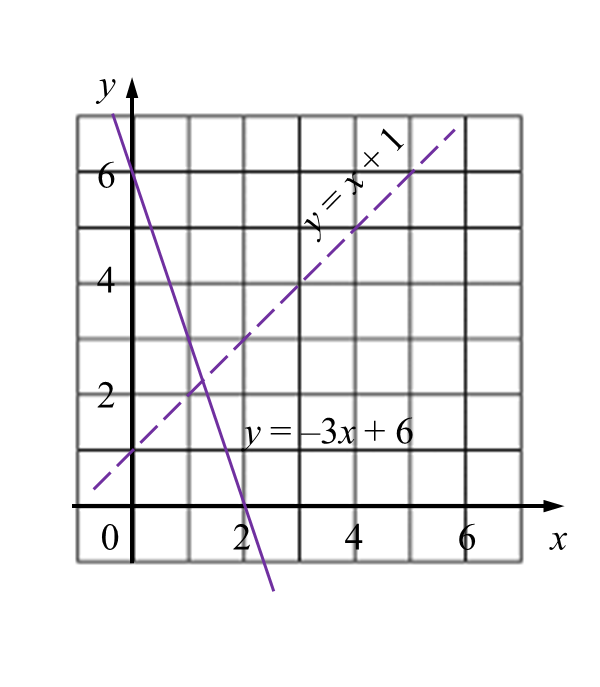# SPM Mathematics 2016, Paper 2 (Questions 1 & 2)

Question 1 (3 marks):
On the graph in the answer space, shade the region which satisfies all three inequalities y ≥ –3x + 6, y > x + 1 and y ≤ 5.$-\frac{2}{3x-5}=\frac{x}{3x-1}$